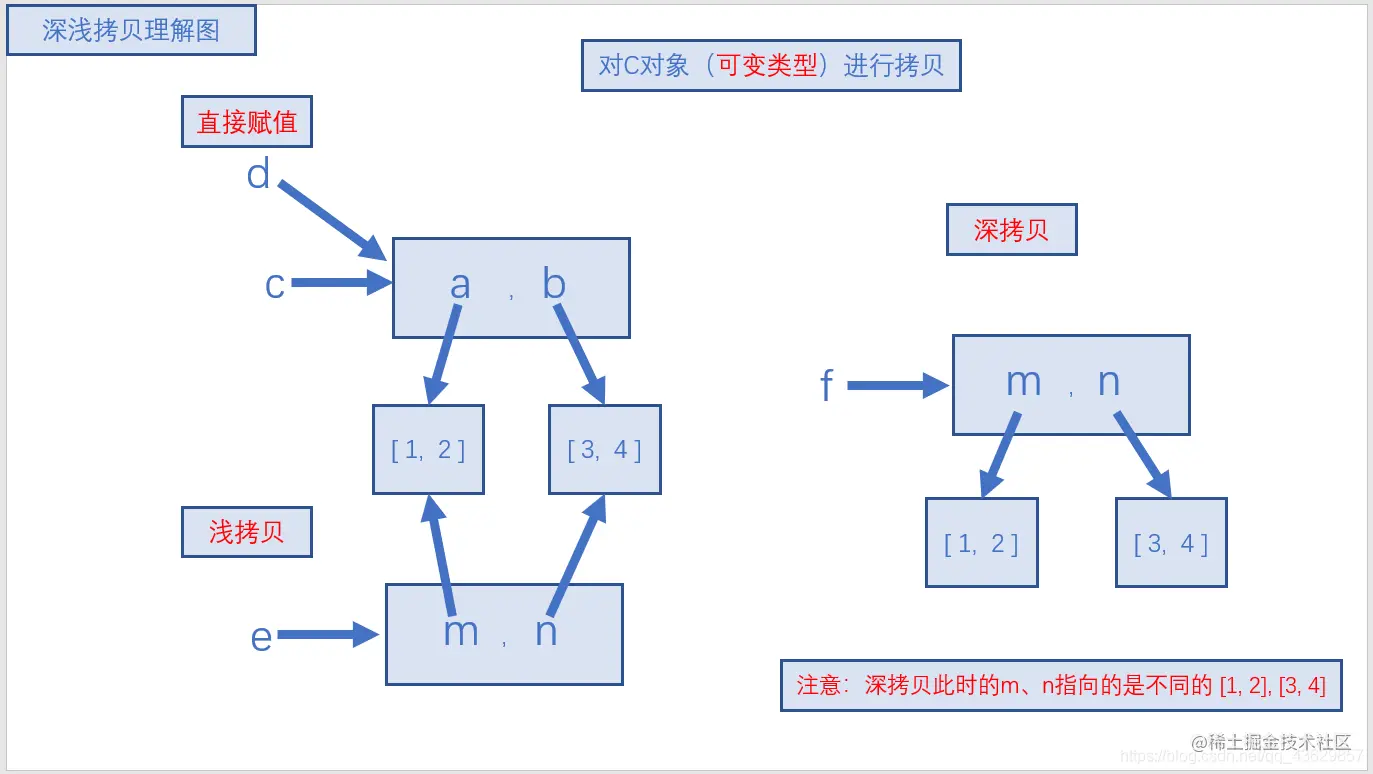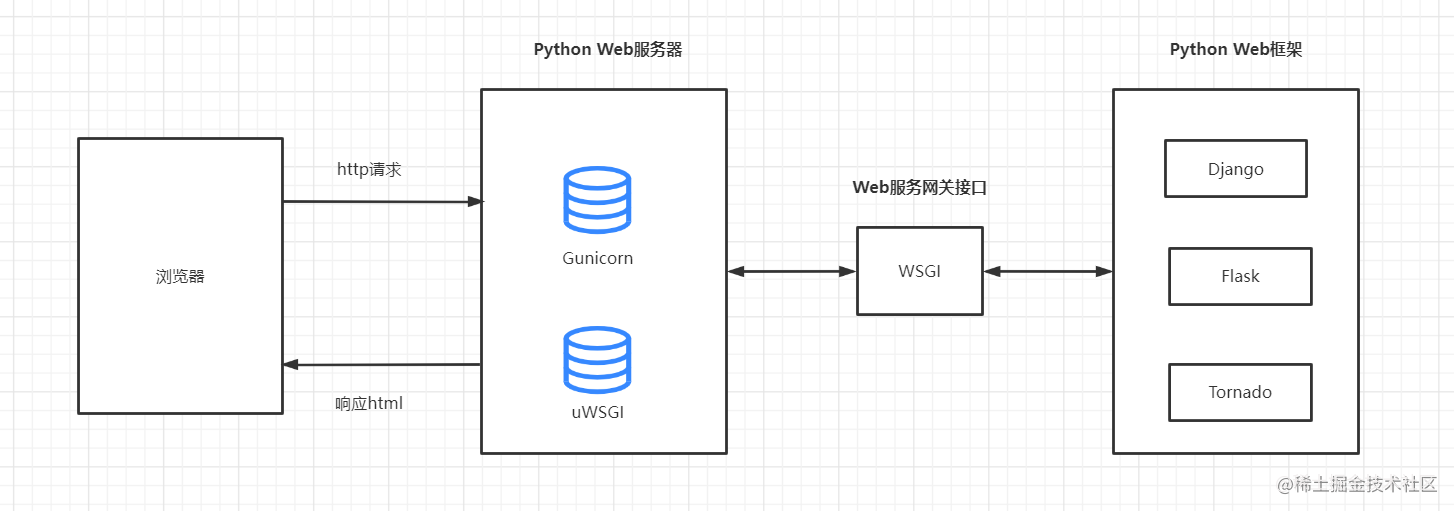# Python 技術要點彙總

## with 語句

`with` 語句適用於對資源進行訪問的場合，確保不管使用過程中是否發生異常都會執行必要的清理操作，釋放資源。底層通過 `__enter__``__exit__`，來實現上下文管理，釋放資源。

```python

file = './a.txt'

with open(file) as f: file_data = file.read() print(file_data)

```

## 閉包、裝飾器

```python def func(number):

``````# 在函式內部再定義一個函式，並且這個函式用到了外部函式的變數，
# 那麼將這個函式以及用到的一些變數稱之為閉包
def func_in(number_in):
print("in func_in 函式, number_in is %d" % number_in)
return number + number_in

# 這裡返回的就是閉包
return func_in
``````

```

```python import time

def calc_time(func): """ 計算函式執行時間 """

``````def wapper():
start_time = time.time()
func() # 呼叫傳遞過來的函式
use_time = start_time - time.time()
print(use_time)

return wapper
``````

@calc_time
def demo():

``````for i in range(100000):
print(i)
``````

```

`@calc_time` 的作用就是會讓 Python直譯器 執行 `demo = calc_time(demo)`

```python

# 1、定義一個帶引數的函式

def router(name):

``````# 2、函式內部定義裝飾器
def _router(func):

def wapper():
pass

return wapper

# 3、返回裝飾器
return _router
``````

```

```python

# 1、定義一個帶引數的函式

def router(path):

``````# 2、函式內部定義裝飾器
def _router(func):

def wapper():
print('path:', path)    # 使用函式傳遞過來的引數
func()

return wapper

# 3、返回裝飾器
return _router
``````

# 使用router裝飾器

@router('/index') def index(): print('首頁')

index()

# 結果如下

path: /index 首頁

```

1. 首先執行 `router('/index')`的函式呼叫，返回了 `_router` 裝飾器
2. 然後就是執行Python的語法糖 `@_router`，把 `index`的函式引用傳遞給 `_router(func)`
3. `index = _router(index)`
4. 最後就是呼叫 `index()` 函式

Python 裝飾器使用詳解 https://juejin.cn/post/6961360227690086437

## 迭代器、生成器

```python class FibIterator(object): """斐波那契數列迭代器"""

``````def __init__(self, n):
"""
:param n: int, 指明生成數列的前n個數
"""
self.n = n

# current用來儲存當前生成到數列中的第幾個數了
self.current = 0

# num1用來儲存前前一個數，初始值為數列中的第一個數0
self.num1 = 0

# num2用來儲存前一個數，初始值為數列中的第二個數1
self.num2 = 1

def __next__(self):
"""被next()函式呼叫來獲取下一個數"""
if self.current < self.n:
num = self.num1
self.num1, self.num2 = self.num2, self.num1+self.num2
self.current += 1
return num
else:
raise StopIteration

def __iter__(self):
"""迭代器的__iter__返回自身即可"""
return self
``````

if name == 'main': fib = FibIterator(10) for num in fib: print(num, end=" ")

# 結果如下

0 1 1 2 3 5 8 13 21 34 ```

Python 生成器 https://juejin.cn/post/6948437559533895693

```python In : L = [i for i in range(10)]

In : G = (i for i in range(10))

In : type(L) Out: list

In : type(G) Out: generator

```

```python def fib(n):

``````cur = 0
num1 = 0
num2 = 1

while cur < n:

yield num1

num1, num2 = num2, num1 + num2

cur += 1
``````

In : f = fib(5)

In : type(f) Out: generator

In : next(f) Out: 0

In : next(f) Out: 1

In : next(f) Out: 1

In : next(f) Out: 2

In : next(f) Out: 3

## In : next(f)

StopIteration Traceback (most recent call last) in ----> 1 next(f)

StopIteration:

In : for i in fib(10): ...: print(i, end=' ') ...: 0 1 1 2 3 5 8 13 21 34 In :

```

```python

import sys import time

def calc_time(func): """ 計算函式執行時間裝飾器 """

``````def wapper(*args, **kwargs):
start_time = time.time()
f = func(*args, **kwargs)
use_time = time.time() - start_time
print(use_time, 's')
return f
return wapper
``````

@calc_time def get_list(n): """列表推導式生成列表資料""" return [i for i in range(n)]

@calc_time def generate_list(n): """通過生成器生成列表資料""" return (i for i in range(n))

n = 1_0000_0000 li = get_list(n) g_li = generate_list(n)

print('li size:', sys.getsizeof(li))

print('g_li size', sys.getsizeof(g_li))

for i in range(5): print('li', li[i], 'g_li', next(g_li))

```

```python 4.615425109863281s # 列表推導式所花費的時間 0.0s # 生成器所花費的時間

li size: 859724472 # 列表推導式產生物件大小 g_li size 120 # 生成器產生物件的大小

li 0 g_li 0 li 1 g_li 1 li 2 g_li 2 li 3 g_li 3

```

```python def read_file(file): with open(file, mode='r') as f:

``````    # f.read() 載入所有資料到記憶體中

yield row   # 通過 yield 返回每行資料
``````

file = 'aaa.txt'

```

## 賦值與深淺拷貝

• 直接賦值： 其實就是指向物件的引用（別名）。
• 淺拷貝(copy)： 拷貝父物件，不會拷貝物件的內部的子物件。但對於不可變資料型別，不會拷貝，僅僅是指向
• 深拷貝(deepcopy)： `copy` 模組的 `deepcopy` 方法，完全（遞迴）拷貝了父物件及其子物件。

```python

# 直接賦值

In : import copy

In : c = [ [1, 2], [3, 4] ]

In : d = c

In : id(d), id(c) Out: (2365035580680, 2365035580680) ```

`d = c` 賦值引用，`c``d` 都指向同一個物件，地址相同。

```python In : # 淺拷貝

In : e = copy.copy(c)

In : id(e), id(c) Out: (2365034915848, 2365035580680)

In : e Out: [[1, 2], [3, 4]]

In : c Out: [[1, 2], [3, 4]]

In : e.append(5)

In : e Out: [[1, 2, 5], [3, 4]]

In : c Out: [[1, 2, 5], [3, 4]]

```

`e = copy.copy(c)` 淺拷貝，`c``e` 是一個 獨立的物件（地址不同），但他們的 子物件還是指向統一物件即引用。因此往`e` 新增資料 5，會影響到 `c`

```python In : # 深拷貝

In : c=[ [1, 2], [3, 4] ]

In : f = copy.deepcopy(c)

In : id(c), id(f) Out: (2365035892552, 2365035662792)

In : c Out: [[1, 2], [3, 4]]

In : f Out: [[1, 2], [3, 4]]

In : f.append(5)

In : f.append(6)

In : f.append(7)

In : c Out: [[1, 2], [3, 4]]

In : f Out: [[1, 2, 6], [3, 4, 7], 5]

```

`f = copy.deepcopy(c)` 深度拷貝， `f` 完全拷貝了 `c` 的父物件及其子物件，兩者是完全獨立的`f` 做任何的修改都不會影響到 `c`• `copy.copy()` 對於可變型別，會進行淺拷貝。
• `copy.copy()` 對於不可變型別，不會拷貝，僅僅是指向。
• `copy.deepcopy()` 深拷貝對可變、不可變型別都一樣遞迴拷貝所有，物件完全獨立。

## GIL鎖

GIL 的全稱為 `Global Interpreter Lock`（全域性直譯器鎖），由於這個鎖的存在，`CPython` 在多執行緒併發的環境下 同一時刻 只有一個執行緒在執行，無法充分利用多核 `CPU` 的效能。雖然很雞肋，但是遇到 `IO` 堵塞的情況下，全域性直譯器鎖會釋放，讓其他執行緒工作。例如：在檔案讀取，網路請求（IO密集的場景下），還是能有效的提升效能。

1、使用多程序

2、使用C語言擴充套件模組

3、選用其他沒有 GIL 的直譯器代替 `CPython`

## 程序，執行緒，協程

• 程序 Process 是作業系統資源分配的基本單位
• 在 Python 中使用 `multiprocessing` 內建模組可以建立程序。
• 在 Python 中使用 `threading` 內建模組可以建立執行緒。
• 協程 CoRoutine 是一種輕量級的使用者態 微執行緒，實現了上下文環境的儲存與切換。
• 簡單來說，執行緒的排程是由作業系統負責，執行緒的睡眠、等待、喚醒的時機是由作業系統控制，開發者無法決定。使用協程，開發者可以自行控制程式切換的時機，可以在一個函式執行到一半的時候中斷執行，讓出 `CPU`，在需要的時候再回到中斷點繼續執行。切換的時機是由開發者來決定。
• 在 Python 中可以使用 `yield` 關鍵字來實現 協程，或者使用第三方庫 `greenlet, gevent`
• Python 3.5版本之後新增了 `async/await` 關鍵字配合 `asyncio` 內建模組可以實現協程和非同步程式設計。

Python 中程序、執行緒、協程的使用請查閱如下連結：

## asyncio 非同步程式設計

`asyncio``Python 3.4`版本引入到標準庫，`Python3.5` 又加入了 `async/await` 特性，能很方便的建立協程以及控制其進行上下文切換。

```python In : async def task(): ...: ...: i = 0 ...: while i < 5: ...: print(i) ...:

In : c Out:

In : type(c) Out: coroutine ```

```python import asyncio

async def task1(): i = 0 while i < 5: print('task1', i) i += 1 await asyncio.sleep(0.1) # await 讓耗時任務自動掛起

async def task2(): i = 0 while i < 5: print('task2', i) i += 1 await asyncio.sleep(0.1)

def main(): loop = asyncio.get_event_loop()

``````tasks = [
]

# 等待所有任務執行完成
print('end')
``````

if name == 'main': main()

```

```python task2 0 task1 0 task2 1 task1 1 task2 2 task1 2 task2 3 task1 3 task2 4 task1 4 end```

## 單例模式的實現

1、重寫類的 `__new__()` 方法

```python class Singleton(object):

``````def __init__(self, name):
self.name = name

def __new__(cls, *args, **kwargs):

if not hasattr(cls, '_instance'):
cls._instance = super().__new__(cls)

return cls._instance
``````

s1 = Singleton('hui') s2 = Singleton('jun')

print(id(s1), id(s2)) print(s1.name, s2.name)

# 結果如下

3097281233736 3097281233736 # 地址一樣 jun jun # 因此操作的是同一個物件 ```

2、使用裝飾器

```python def singleton(cls):

``````_instance = {}

def _singleton(*args, **kwargs):

if cls not in _instance:

_instance[cls] = cls(*args, **kwargs)

return _instance[cls]

return _singleton
``````

@singleton class Demo(object):

``````def __init__(self, name, age=18):
self.name = name
self.age = age
``````

d1 = Demo('hui', age=18) d2 = Demo('jun', age=21)

print(id(d1), id(d2)) print(d1.name, d1.age) print(d2.name, d2.age)

# 結果如下

2107174554696 2107174554696 hui 18 hui 18 ```

```python

def singleton(cls):

``````print(type(cls))

_instance = {}

def _singleton(*args, **kwargs):

print(args)
print(kwargs)

if cls not in _instance:

print(cls.__dict__)
instance = cls(*args, **kwargs)
print(type(instance))

_instance[cls] = instance

return _instance[cls]

return _singleton
``````

@singleton class Demo(object):

``````def __init__(self, name, age=18):
self.name = name
self.age = age
``````

d1 = Demo('hui', age=18) d2 = Demo('jun', age=21)

print(id(d1), id(d2)) print(d1.name, d1.age) print(d2.name, d2.age) ```

```python # Demo類物件(cls)的型別 ('hui',) # Demo 初始化時的位置引數 {'age': 18} # Demo 初始化時的關鍵字引數

# Demo類物件的__dict__屬性

{'module': 'main', 'init': , 'dict': , 'weakref': , 'doc': None }

# 通過類物件構造出的例項物件

('jun',) {'age': 21} 2447199767752 2447199767752 # 地址相同

# 所以後面建立物件所傳遞的初始化引數沒有效果

hui 18 hui 18 ```

## CGI，WSGI

`CGI` 全稱為 `Common Gateway Interface` （通用閘道器介面），是一種定義程式和伺服器互動方式的標準協議。

`WSGI`的全稱為 `Python Web Server Gateway Interface` （Web 服務閘道器介面），它是Web伺服器和Web應用程式通訊的介面規範，用來支援Web伺服器和Web應用程式互動。## Python 記憶體管理與垃圾回收機制

Python中的記憶體管理由Python私有堆空間管理。所有Python物件和資料結構都位於私有堆中。程式設計師無權訪問此私有堆。Python直譯器負責處理這個問題。

Python 記憶體管理機制：引用計數、垃圾回收、記憶體池。

「其他文章」